# 12. Find all solutions with 0 <I<27: sec r = -2 13. Find all real solutions:...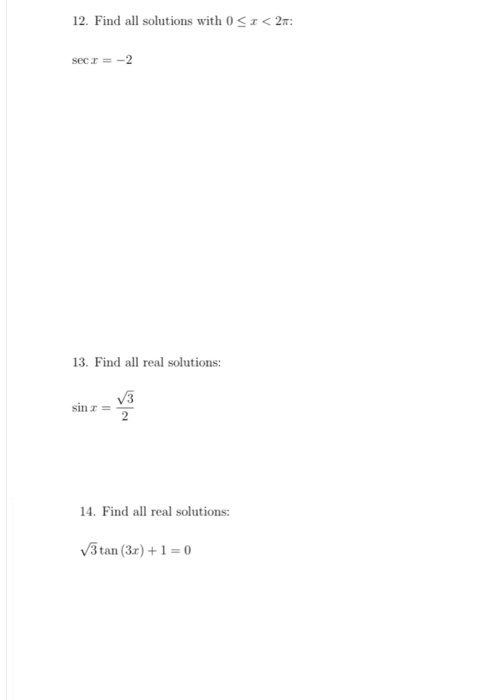12. Find all solutions with 0 <I<27: sec r = -2 13. Find all real solutions: sin r 2 14. Find all real solutions: 3 tan (3x) + 1 = 0

This Homework Help Question: "12. Find all solutions with 0 <I<27: sec r = -2 13. Find all real solutions:..." No answers yet.

We need 10 more requests to produce the answer to this homework help question. Share with your friends to get the answer faster!

0 /10 have requested the answer to this homework help question.

Once 10 people have made a request, the answer to this question will be available in 1-2 days.
All students who have requested the answer will be notified once they are available.

#### Earn Coin

Coins can be redeemed for fabulous gifts.

Similar Homework Help Questions
• ### 8. For 0 < < 27. find all solutions of sec r = eser. 14. Given...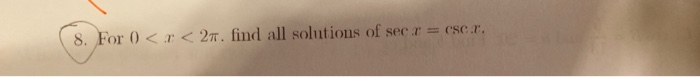8. For 0 < < 27. find all solutions of sec r = eser. 14. Given a right triangle with sides a and b and hypotenuse c. 20. Find b and c 1 sin B and a = 2 B! tan A 100 and 6 = 100. Find a and c. C. cos B = 5 13 and a = 20. Find b and c. 15. Find two values of a between 0 and 2 such that tanx = V3....

• ### Find all solutions to 2 sin(0) = V3 on the interval 0 < 0 < 27...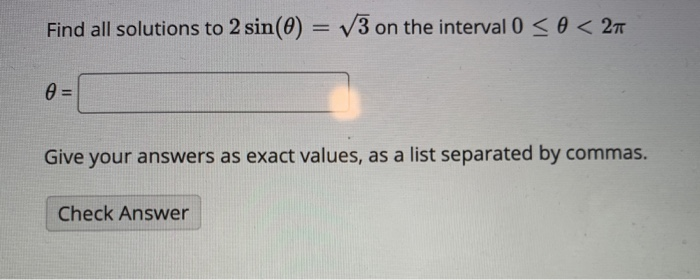Find all solutions to 2 sin(0) = V3 on the interval 0 < 0 < 27 0 = Give your answers as exact values, as a list separated by commas. Check Answer

• ### Find all real solutions of the equation (2 – 6)? = 4. 21 = and 12...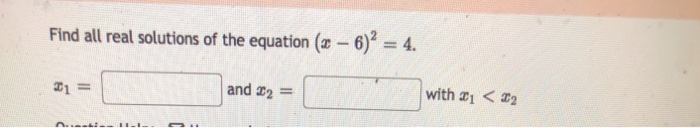Find all real solutions of the equation (2 – 6)? = 4. 21 = and 12 with i < 22

• ### can you help me with 12-17 please (i dont need 18) 1 . - < <...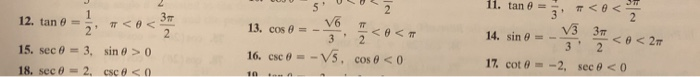can you help me with 12-17 please (i dont need 18) 1 . - < < 27 12. tan 8 = 5, +<o<- 15. sec @ = 3, sin 8 > 0 14. sin 0 = - 3.3< 17 cote = -2, sec @ < 0 16. csc 8 --V5, cos e < 0

• ### 2. Solve 2 sec @ + tan 0 = 2 cose, 050<21. 3. Solve cos 2x...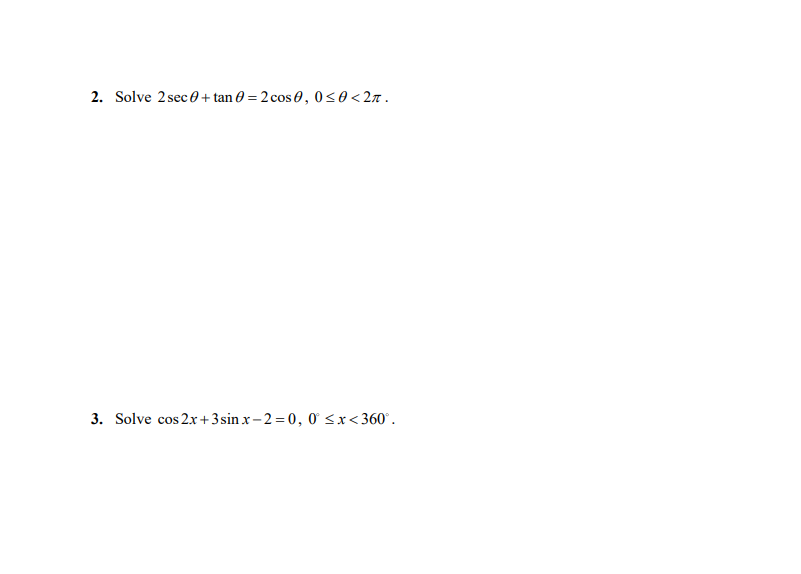2. Solve 2 sec @ + tan 0 = 2 cose, 050<21. 3. Solve cos 2x + 3 sin r-2=0, 0 <x<360°.

• ### Solve 5 sin(20) + 6 cos(0) = 0 for all solutions 0 = 0 < 27...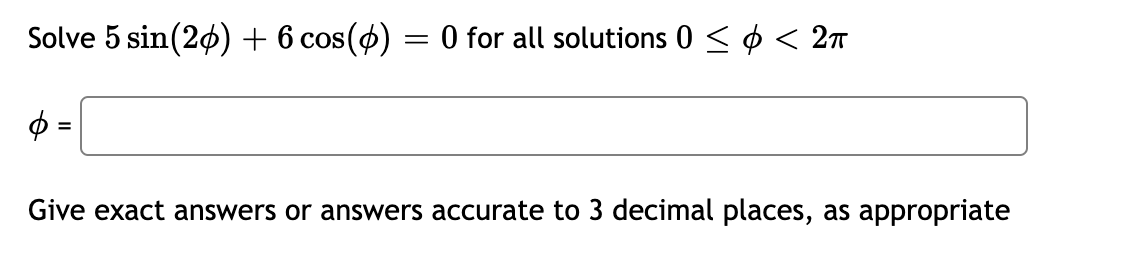Solve 5 sin(20) + 6 cos(0) = 0 for all solutions 0 = 0 < 27 Give exact answers or answers accurate to 3 decimal places, as appropriate

• ### . c) + < 2 b) 2 + 3x 27, 0. Solve for r: r' +.... c) + < 2 b) 2 + 3x 27, 0. Solve for r: r' + 2.r < 2.1? +12

• ### 19. If the cos u= -5/13 where it <u<37 12 and sin v= 8/15 where tan...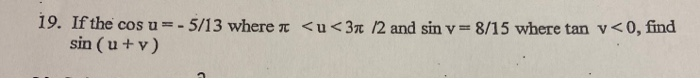19. If the cos u= -5/13 where it <u<37 12 and sin v= 8/15 where tan v<0, find sin (u+v)

• ### 14. 4 -/1 points McKTrig8 6.2.037. Find all degree solutions in the interval 0° SO <...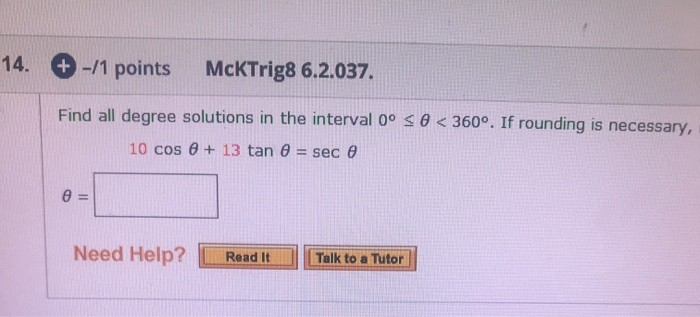14. 4 -/1 points McKTrig8 6.2.037. Find all degree solutions in the interval 0° SO < 360°. If rounding is necessary, 10 cos + 13 tan 6 = sec Need Help? Read It Talk to a Tutor

• ### Find all solutions to cos(7a) - cos(a) = sin(4a) on 0 Sa<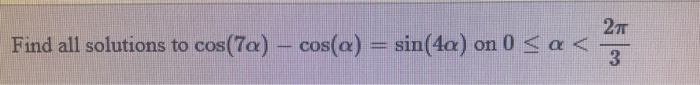Find all solutions to cos(7a) - cos(a) = sin(4a) on 0 Sa<